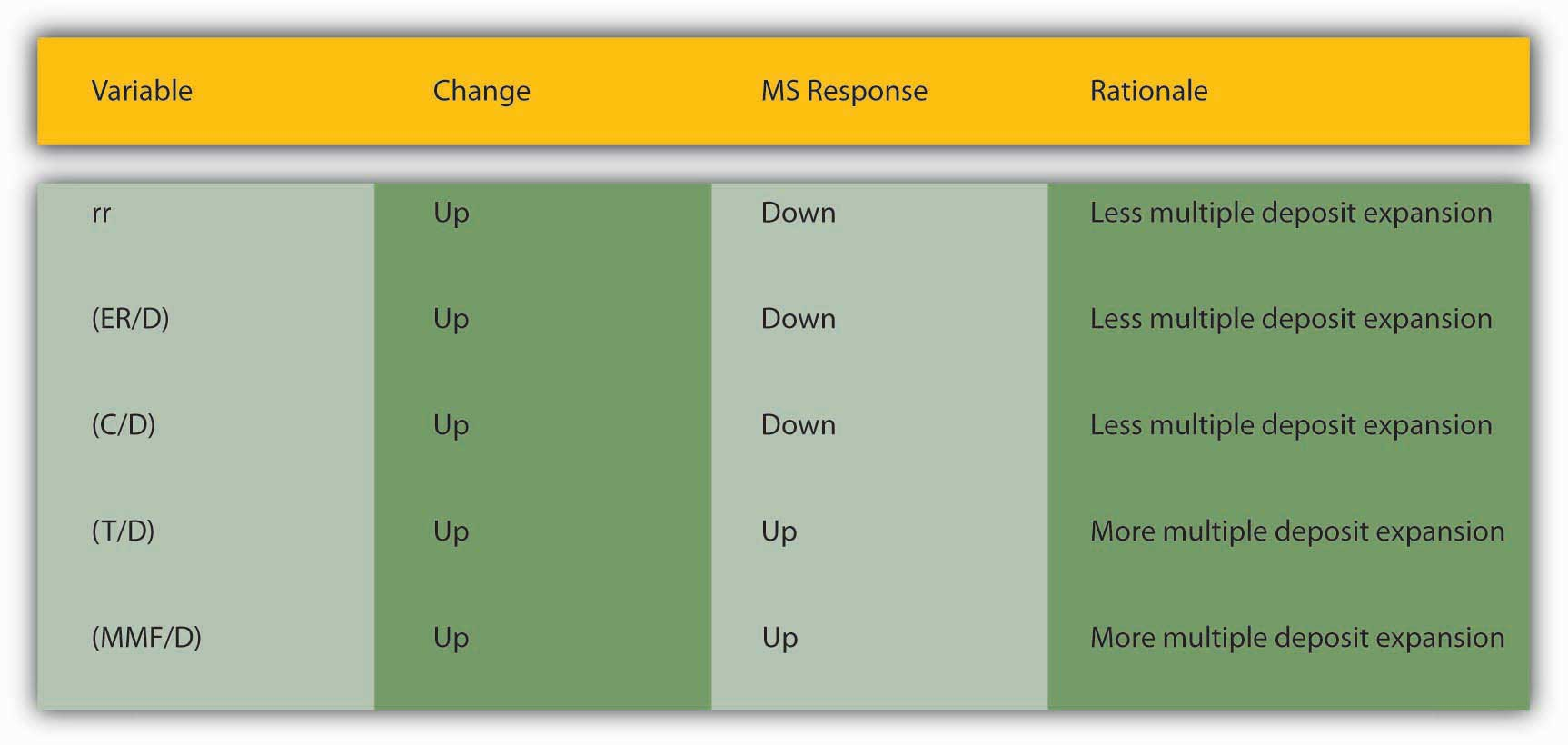# 15.6: Summary and Explanation

•• Contributed by No Attribution by request
• Anonymous by request

learning objective

1. How do central banks, banks, depositors, and borrowers influence the money supply?

By way of summary, Figure 15.6 "Major influences on m" explains why each of the major variables influences m1 and m2 in the ways implied by the equations presented above.

Figure 15.6 Major influences on m1 and m2As we saw at the beginning of this chapter, currency holdings, excess reserves, and required reserves slow down the multiple deposit creation process by removing funds from it. The bigger rr and ER/D are, the less each bank lends of the new deposits it receives. The bigger C/D is, the less money is deposited in the first place. For those reasons, we place those variables in the denominator. The larger the denominator, holding the numerator constant, the smaller m1 or m2 will be, of course. The appropriate money supply components compose the numerator-currency, and checkable deposits for m1, and currency, checkable deposits, time deposits, and money market mutual funds compose the numerator for m2.

This leaves us to consider why C/D, rr, and ER/D change over time. Short term, the currency ratio varies directly with the interest rate and the stability of the banking system. As the interest rate increases, the opportunity cost of keeping cash increases, so people are less anxious to hold it. People are also less anxious to hold currency if the banking system is stable because their money is safer in a checking deposit. If interest rates are extremely low or people believe the banks might be shaky, they naturally want to hold more physical cash. Longer term, C/D may be influenced by technology and loophole mining, encouraging bankers and depositors to eschew traditional checkable deposits in favor of sweep accounts.

The required reserve ratio is mandated by the central bank but, as noted in Chapter 10 "Innovation and Structure in Banking and Finance", loophole mining and technology have rendered it less important in recent years because sweep accounts allow banks to minimize the de jure level of their checkable deposits. In many places, rr is no longer a binding constraint on banks so, as we’ll see, most central banks no longer consider changing it as an effective monetary policy tool. (This in no way affects the money multiplier, which would provide the same figure for m1 or M2 whether we calculate them as above or replace rr and ER/D with R/D, where R = total reserves.)

# Stop and Think Box

Prove the assertion made above: “This in no way affects the money multiplier, which would provide the same figure for m1 or M2 whether we calculate them as above or replace rr and ER/D with R/D, where R = total reserves.” Suppose that C = 100, R = 200, and D = 500 and that R is composed of required reserves of 100 and excess reserves of 100. That means that rr must equal .2(100/500).

Under the formula provided in the text,

$m 1 = \dfrac{1 + ( C / D )}{[ r r + ( E R / D ) + ( C / D ) ]}m 1 = 1 + .2 / .2 + .2 + .2 = 1.2 / .6 = 2.0$

Under the formula suggested above,

$m 1 = \dfrac{1 + ( C / D )}{ ( R / D ) + ( C / D ) } m 1 = 1.2 / .4 + 2 = 2.0$

Excess reserves (or just reserves in a system without required reserves) are inversely related to the interest rate. In the early 1960s and early 2000s, when the interest rate was well less than 5 percent, ER/D was high, at .003 to .004. In the 1970s and 1980s, when interest rates were 10 percent and higher, ER/D dropped to .001 to .002. As we learned in Chapter 9 "Bank Management", expected deposit outflows directly affect excess reserve levels as banks stock up on reserves to meet the outflows. When uncertainty is high or a banking crisis is in progress or appears imminent, bankers will increase ER to protect their banks.

In summary, the central bank influences the money supply by controlling the monetary base and, to a far lesser extent, the required reserve ratio. Depositors, banks, and borrowers influence the money supply by influencing m1 and M2, specifically by determining the money multiplier, with depositors largely in control of C/D; depositors and banks interacting via deposit outflow expectations to determine E/R; and borrowers, depositors, banks, and the central bank interacting to determine interest rates and hence to some extent both C/D and E/R.

key takeaways

• Central banks control MB and rr, and affect interest rates, which in turn affect C/D and ER/D.
• Depositors determine C/D by deciding how much cash versus deposits to hold. They also influence interest rates.
• Banks influence interest rates and determine ER/D by deciding how many excess reserves to hold in the face of expected deposit outflows and interest rates.
• Borrowers influence the interest rate and hence to some extent C/D and ER/D.